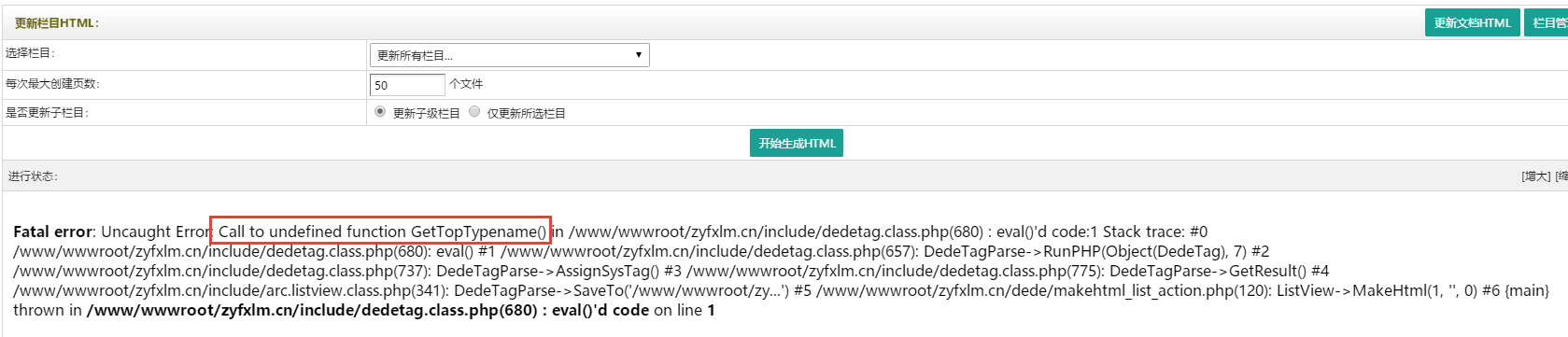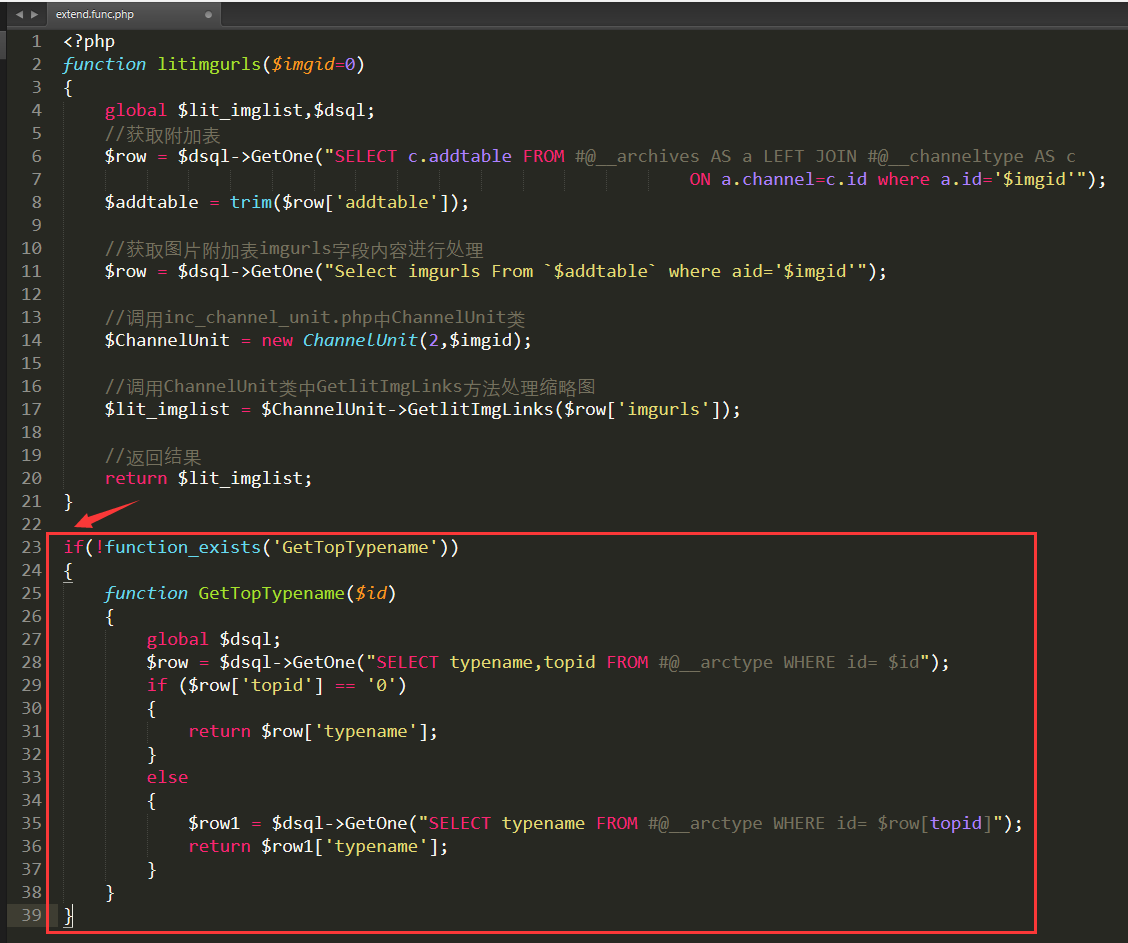if(!function_exists('GetTopTypename'))
{
function GetTopTypename(\$id)
{
global \$dsql;
\$row = \$dsql->GetOne("SELECT typename,topid FROM dede_arctype WHERE id= \$id");
if (\$row['topid'] == '0')
{
return \$row['typename'];
}
else
{
\$row1 = \$dsql->GetOne("SELECT typename FROM dede_arctype WHERE id= \$row[topid]");
return \$row1['typename'];
}
}
}